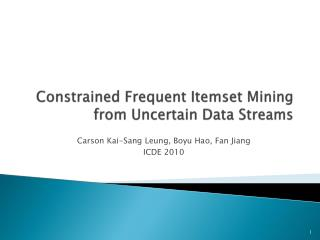Download PresentationConstrained Frequent Itemset Mining from Uncertain Data Streams

# Constrained Frequent Itemset Mining from Uncertain Data Streams - PowerPoint PPT PresentationDownload Presentation## Constrained Frequent Itemset Mining from Uncertain Data Streams

- - - - - - - - - - - - - - - - - - - - - - - - - - - E N D - - - - - - - - - - - - - - - - - - - - - - - - - - -
##### Presentation Transcript

1. Constrained Frequent Itemset Miningfrom Uncertain Data Streams Carson Kai-Sang Leung, BoyuHao, Fan Jiang ICDE 2010

2. outline • Motivation • Method (UF-streaming+, UF-streaming*, CUF-streaming) • Experimental results • Conclusion

3. motivation • There are many situations in which ones are uncertain about the contents of transactions. Moreover, there are also situations in which users are interested in only some portions of the mined frequent itemsets.

4. Method--UF-streaming+ Minsup=1.2preMinsup=0.9 First batch: a b c d e 1.8 1.6 1.9 0.9 1.4 例如: expSup({a, e}) = (1 × 0.9 × 0.6) + (1 × 0.9 × 0.7)= 1.17 ≥ preMinsup expSup({c, e}) = (1 × 0.7 × 0.6)+ (1 × 0.8 × 0.7) = 0.98 ≥ preMinsup expSup({d, e}) =1 × 0.9 × 0.1 = 0.09 < preMinsup expSup({a, c, e})= (1 × 0.9 × 0.7×0.6) + (1 × 0.9 × 0.8×0.7) ≈ 0.88 <preMinsup.)

5. Method--UF-streaming+ First batch: {a}{a, c}{a, e}{b}{c}{c, e}{d}{e} 1.8,1.35,1.17,1.6,1.5,0.98,0.9,1.4 ----------------------------------- Second batch: {a} {a, c} {b} {b, d} {c} {d} 0.9, 0.9, 1.4, 1.4, 1.8, 2.0

6. Method--UF-streaming+ Second batch: {a} {a, c} {b} {b, d} {c} {d} 0.9, 0.9, 1.4, 1.4, 1.8, 2.0 ----------------------------- third batch: {a} {a, c} {b} {b, d} {c} {d} 1.7, 1.53, 1.0, 1.0, 1.9 1.2post-processing step: {a}:2.6, {a, c}:2.43,{b}:2.4 and {c}:3.7 satisfying C1.

7. Method--UF-streaming* • the algorithm first usesthe same UF-growth mining technique to find all “frequent”itemsets, and it then checks the mined itemsets against userspecifiedconstraints before storing the constrained itemsetsinthe UF-stream structure. •  • 

8. Method-- CUF-streaming Type1:ANTI-MONOTONE CONSTRAINT min(X.attr) ≥ const  R+ (Xi.attr≤ Xi+1.attr) max(X.attr) ≤ const R- (Xi.attr≥Xi+1.attr) Ex : C1 ≡ min(X.WBC) ≥ 10*103/μL  (e , d , c , b , a ) 9.0 9.5 10.5 11.0 11.5 Type2:MONOTONE CONSTRAINT max(X.attr) ≥ const  R- min(X.attr) ≤ const  R+ Ex: C2 ≡ max(X.RBC) ≥ 6.1 × 106/μL 

9. Type3:CONVERTIBLE ANTI-MONOTONE CONSTRAINTavg(X.attr) ≥ const or sum(X−.attr) ≥ const  R- avg(X.attr) ≤ const or sum(X+.attr) ≤ const R+ Ex: C3 ≡ sum(X.Rainfall ) ≤200mm  Type4:CONVERTIBLE MONOTONE CONSTRAINT sum(X+.attr) ≥ const  R- sum(X−.attr) ≤ const  R+ Ex: C4 ≡sum(X.Rainfall) ≥ 200mm 

10. C1 ≡ min(X.WBC) ≥ 10000/μL R+ (e,d,c,b,a)  (c,b,a) check {a}{a, c} {a, e} {b}{c} {c, e}{d} {e}  {a}, {a, c}, {b} ,{c}

11. Experimental results

12. conclusion • we proposed three tree-based algorithms—namely, UF-streaming+, UF-streaming∗ and CUF-streaming—which integrate : (i) mining of uncertain data (ii) constrainedmining (iii) mining of data streams. These algorithmseffectively mine constrained frequent itemsets from uncertaindata streams.